MATLAB教程（1） MATLAB 基础知识

7 篇文章 16 订阅

https://cn.mathworks.com/help/matlab/learn_matlab/desktop.html

1.启动MATLAB后，桌面会显示默认布局，主要有以下三个部分：

• 当前文件夹
• 命令行窗口
• 工作空间

2.在MATLAB中一般需要创建变量和调用函数。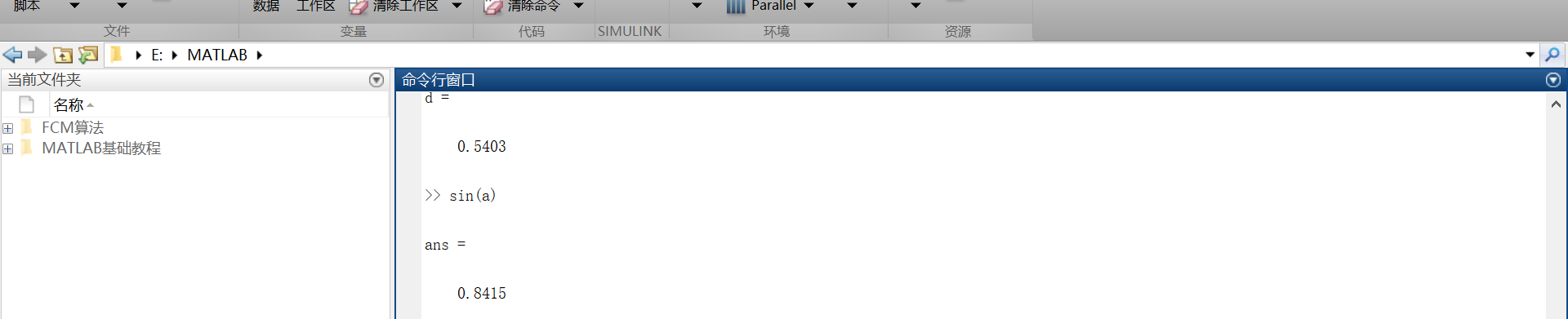3.如果语句以分号结束，表示MATLAB内部会执行这一运算，但是命令行不对计算结果进行输出。

https://cn.mathworks.com/help/matlab/learn_matlab/matrices-and-arrays.html

1.数组的创建

• 在一个单行中创建一个拥有四个元素的数组，其各个元素之间用逗号或者空格隔开。
例如：
a = [1 2 3 4]
这种数组叫做单行矩阵（行向量）。
• 创建一个多行矩阵，其各个行之间用分号隔开。
例如：
b = [1,2;3,4;5,6]
• 还可以用函数来创建矩阵。
例如：创建一个5行1列每一个元素都是0的列向量。
z = zero(5,1)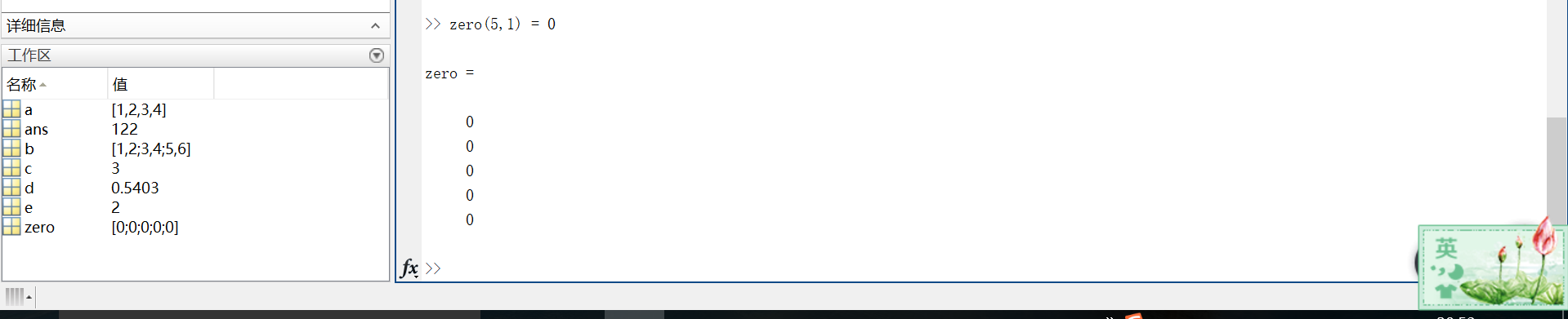2.矩阵和数组的操作

• 在MATLAB中，允许用一个单一的运算或者函数对矩阵中所有的数据进行处理（操作）。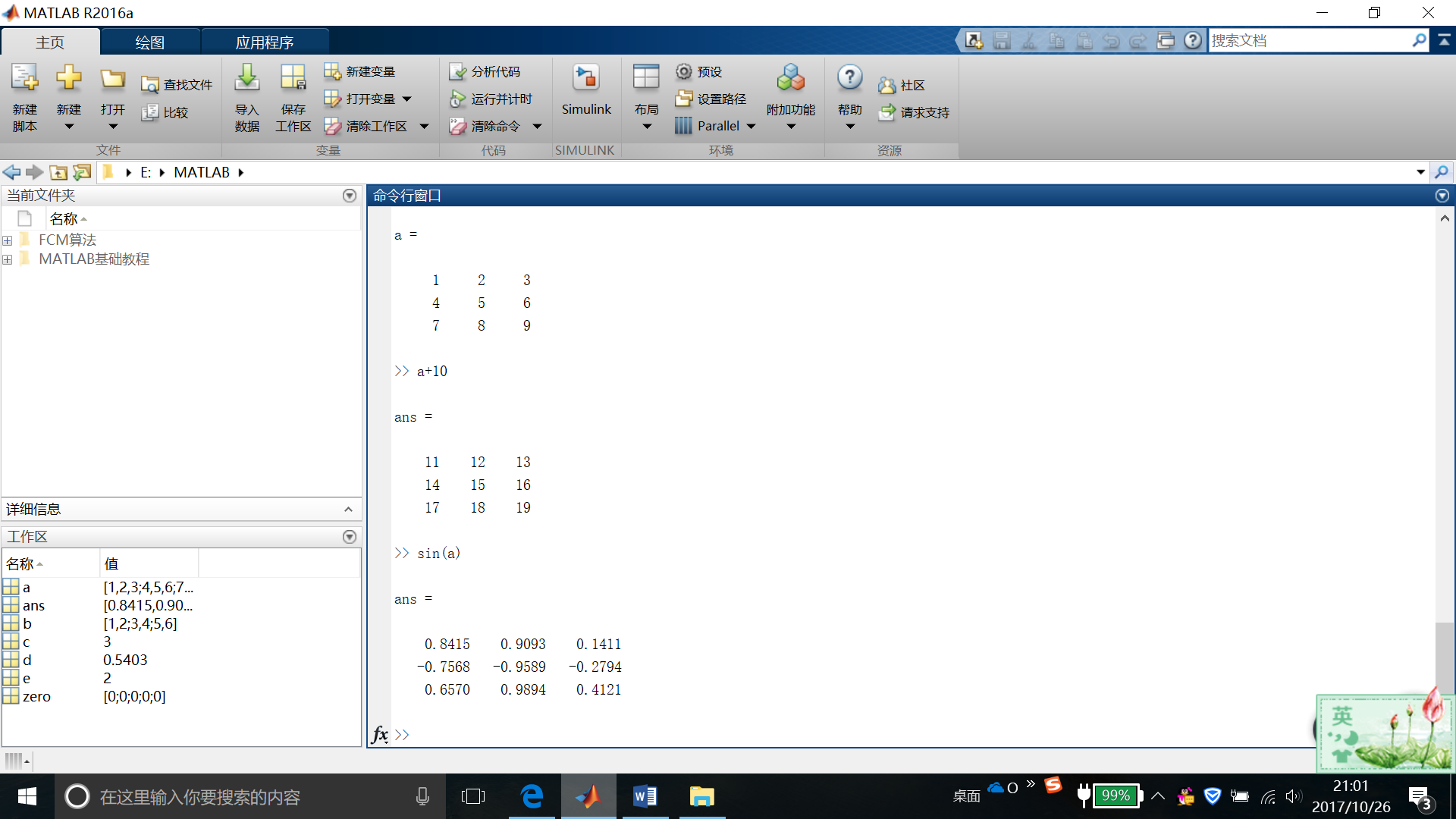• 如果要对矩阵进行转置，则使用单引号(‘)
例如：a’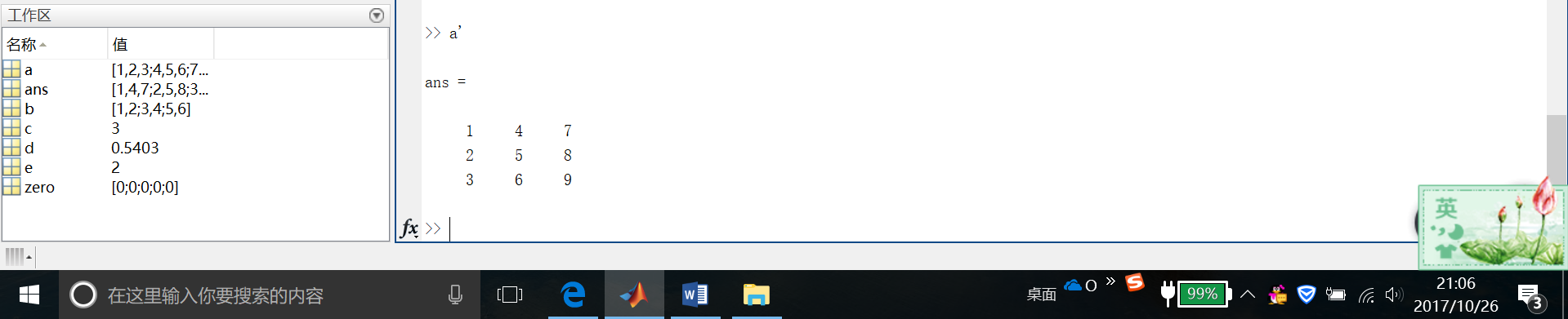• 可以通过执行标准矩阵乘法，通过*运算符来计算内积。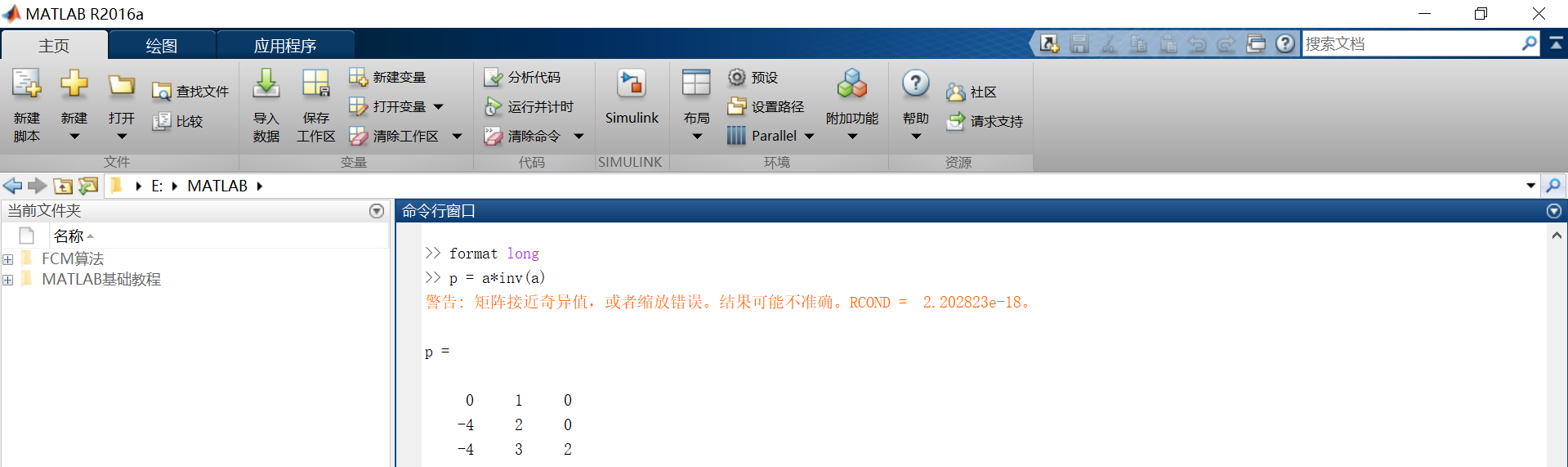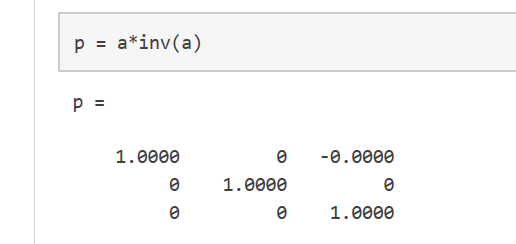format long
p = a*inv(a)

• x = a.*a用来表示对矩阵的每一个元素做平方操作。
若需要对每一个元素做立方运算，则用x = a.^3(如图)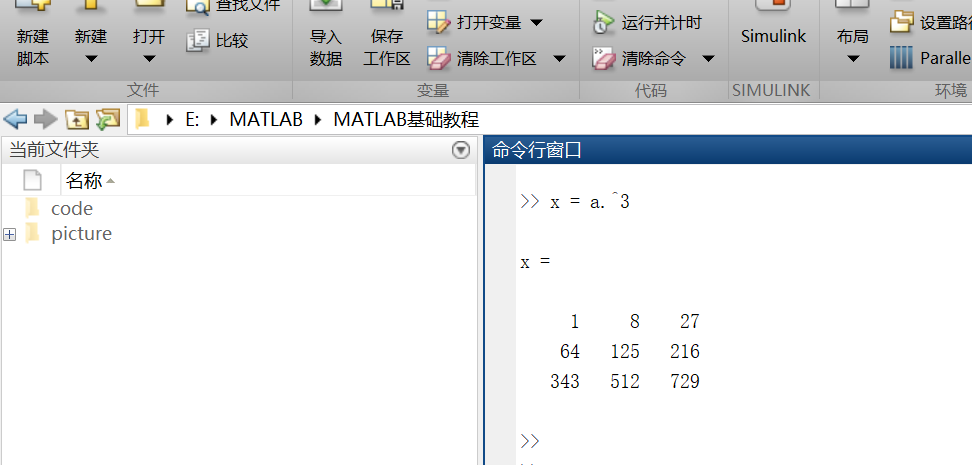• 矩阵的扩充
行方向上：A = [a,a]
列方向上：A = [a; a]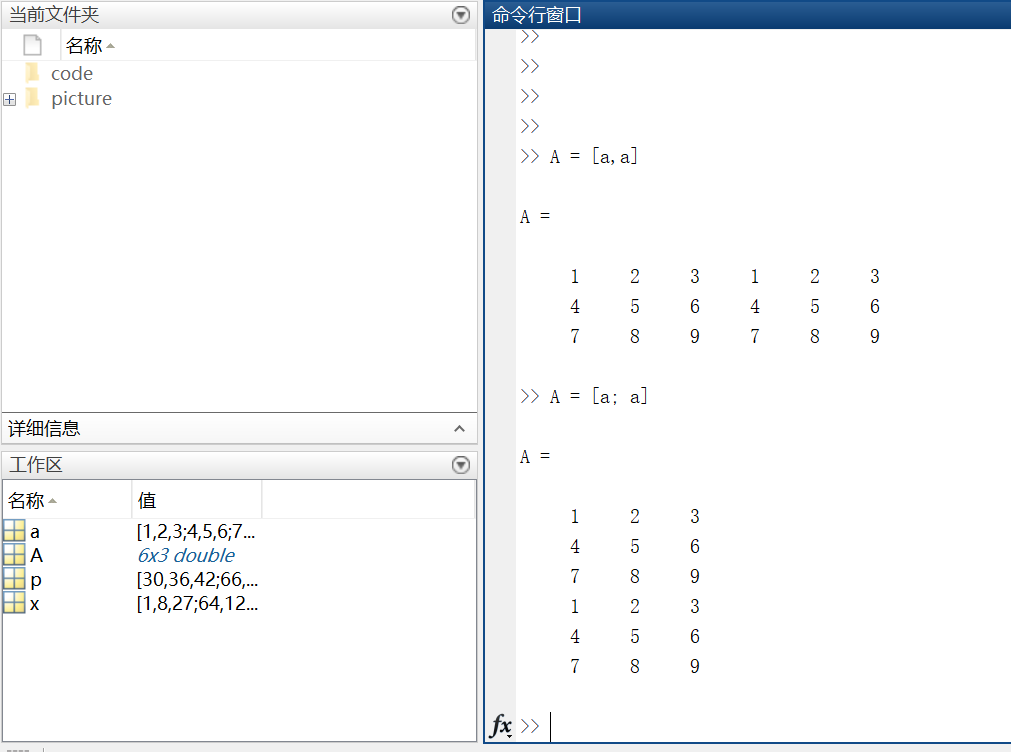• 复数
复数由实部和虚部组成，它的虚部是-1的平方根。
即sqrt(-1) = 0.0000 + 1.0000i
一般用i或者j来代表复数的虚部部分。

https://cn.mathworks.com/help/matlab/learn_matlab/array-indexing.html

MATLAB中的每一个变量都是可以存储多个值的数组。如果想要使用数组中的某一个值，则需要用到索引。

1.单个元素的索引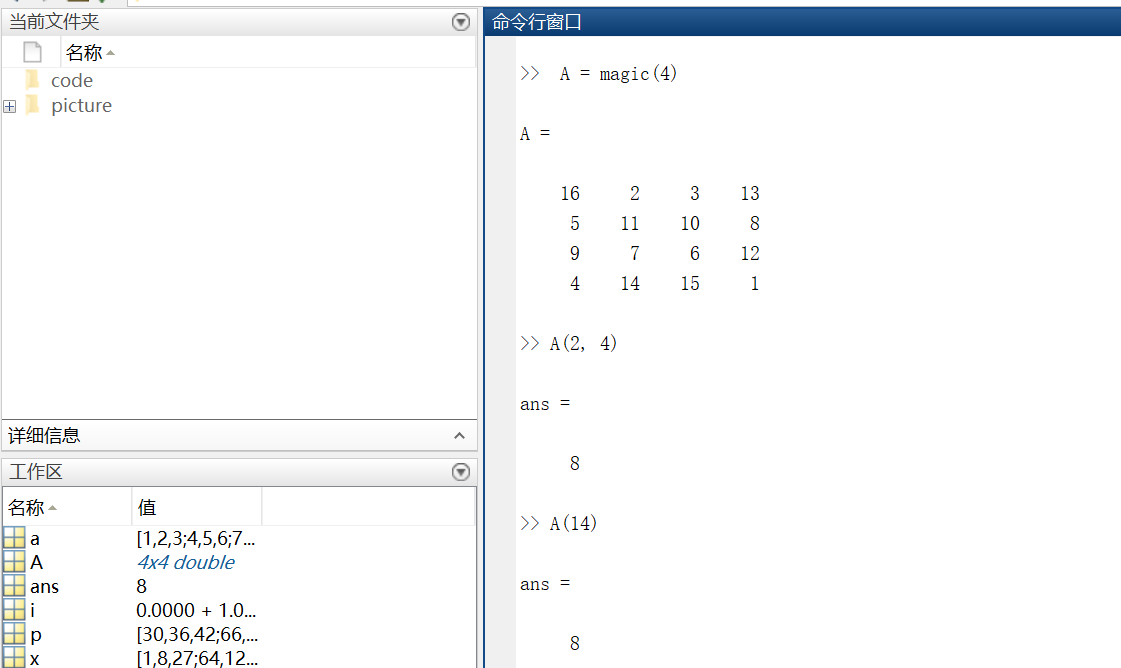2.多个元素的索引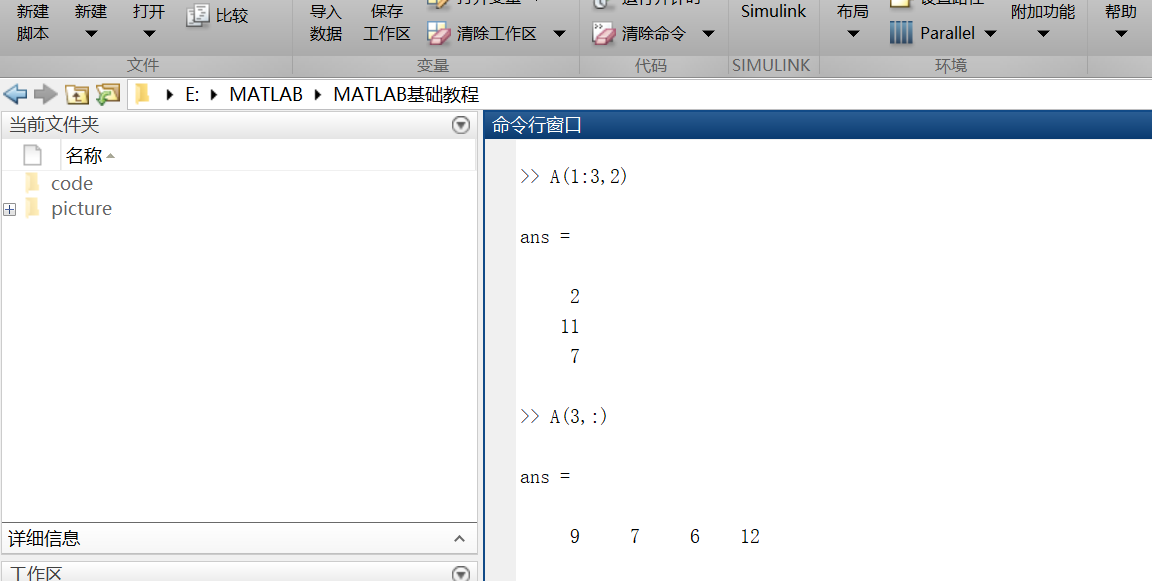B = 0:10:100
—->B = 0 10 20 30 40 50 60 70 80 90 100

https://cn.mathworks.com/help/matlab/learn_matlab/workspace.html

A = magic(4)
B = rand(3,5,2)

• 我们可以通过whos来看工作空间的内容。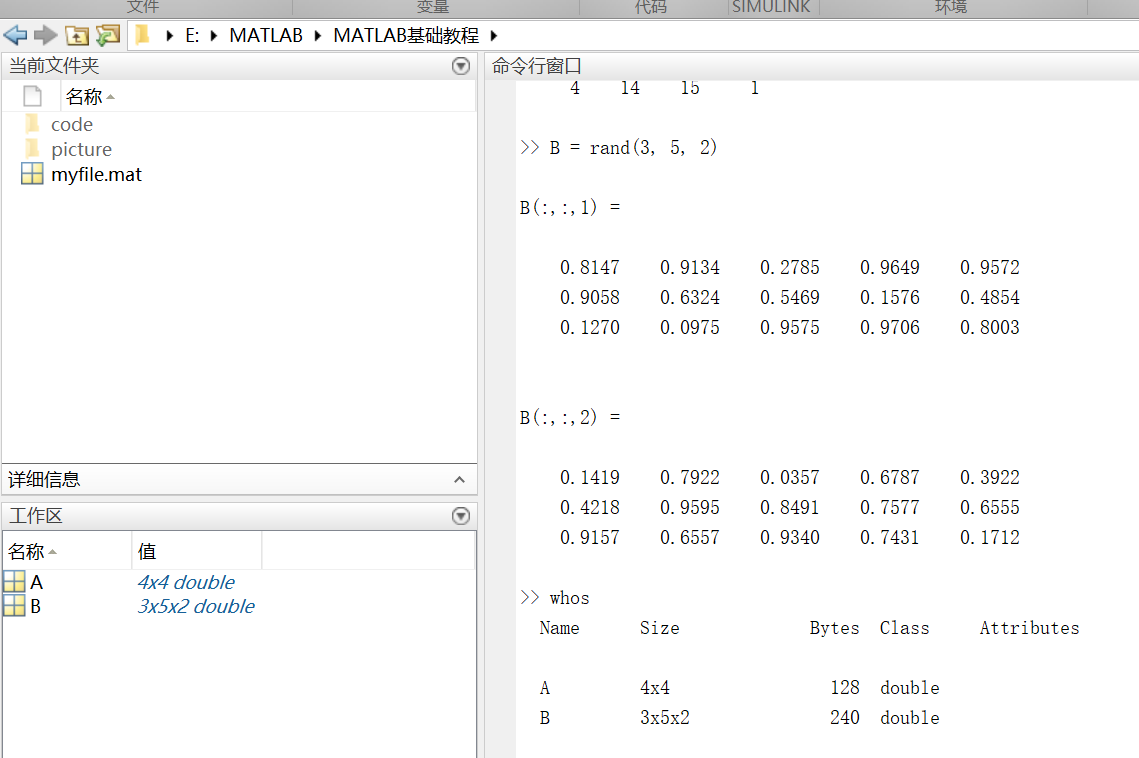• 工作区的变量在退出MATLAB后就不存在了。
所以一般使用save myfile.mat命令来保存工作区变量以便以后使用。
保存操作将当前工作文件夹中的工作区保存在一个压缩文件中，该文件的扩展名为.MAT，称为MAT文件。
• 如果想要清空工作空间里的所有变量，则需使用clear命令

https://cn.mathworks.com/help/matlab/learn_matlab/character-strings.html

• 在处理文本时，将其中的字符序列用单引号括起来，可以将文本分配给变量。
例如：myText = ‘Hello, world’;
• 如果文本本身包括一个单引号，则在定义时使用两个单引号。
例如：otherText = ‘You”re right’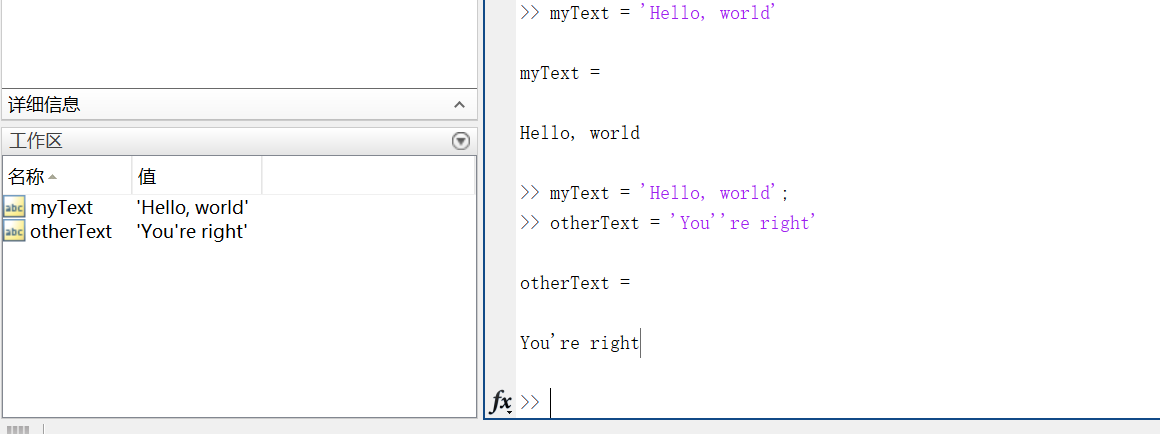• 要将数字转换成字符，就要用到函数。例如num2str()或者int2str()。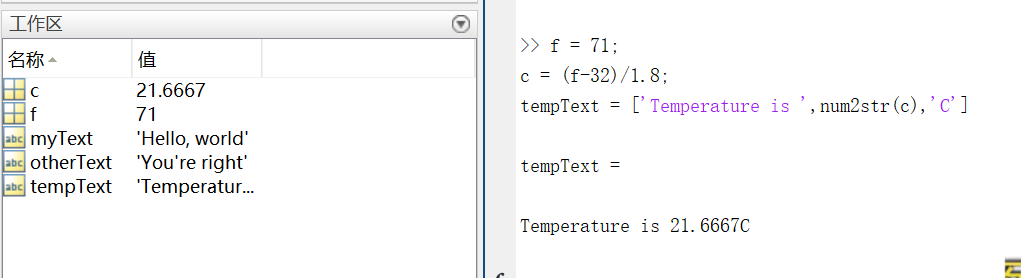https://cn.mathworks.com/help/matlab/learn_matlab/calling-functions.html

MATLAB提供了大量函数来执行计算任务，函数相当于其他编程语言中的子程序或方法。

• 将输入参数用括号括起来，如果有多个参数，则将参数用逗号隔开。
一般通过将函数值赋给某个变量这种方式来返回（输出）函数值，如果有多个输出参数，则需将它们用方括号括起来。
例如：
A = [1 3 5];
[maxA,location] = max(A)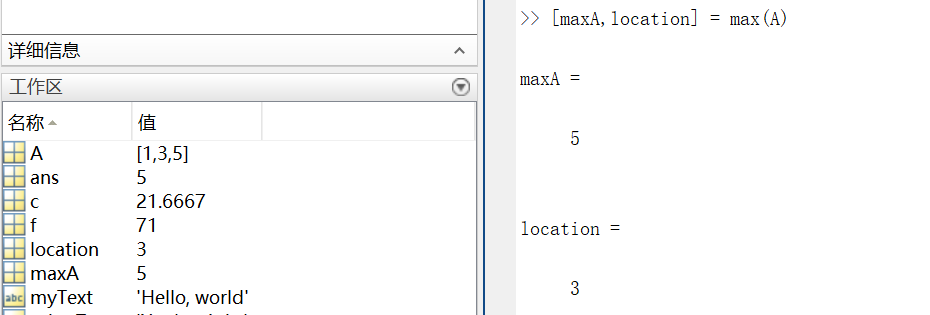• 可以用单引号括住任何字符进行输入。
例如：
disp(‘hello world’)
===>输出 hello world

disp()用来显示变量的内容,括号里可以是字符,结构体,矩阵等等，类似于c语言的printf()。

• clc函数被用来清空命令行。

https://cn.mathworks.com/help/matlab/learn_matlab/plots.html

• 折线图

（1） 画图

x = 0:pi/1000:2*pi;
y = sin(x);
plot(x,y)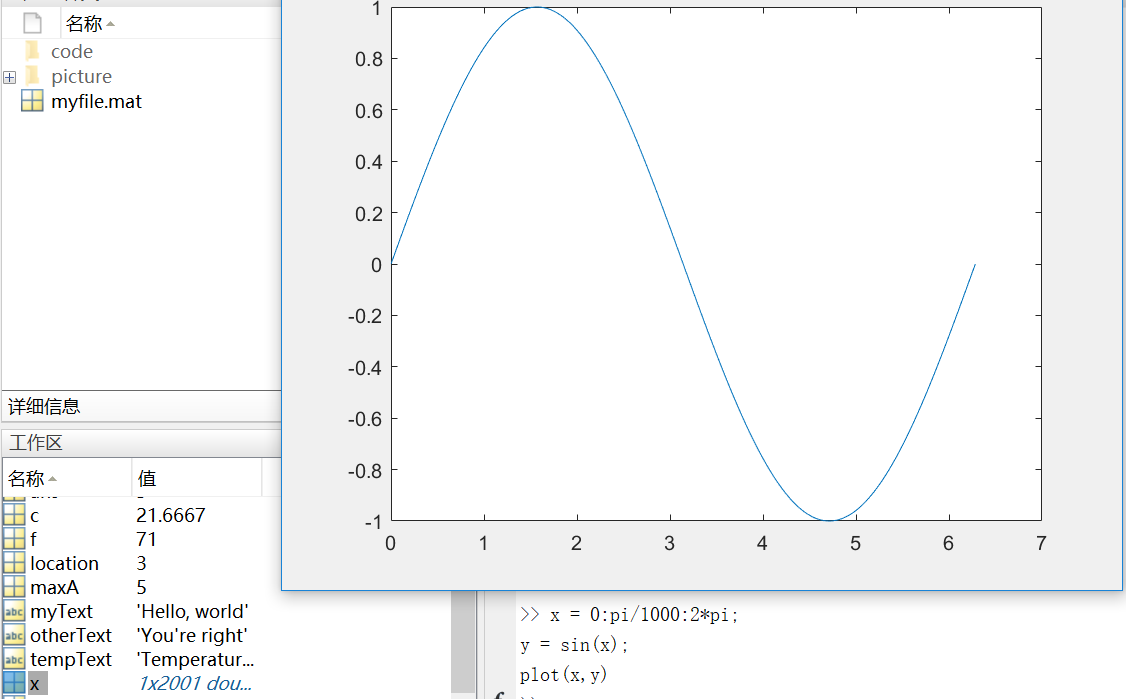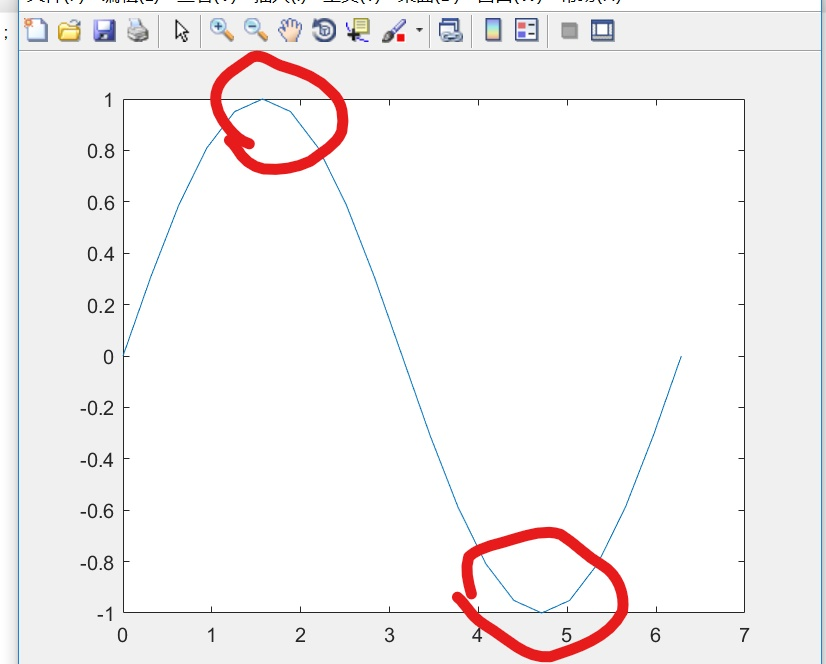（2） 坐标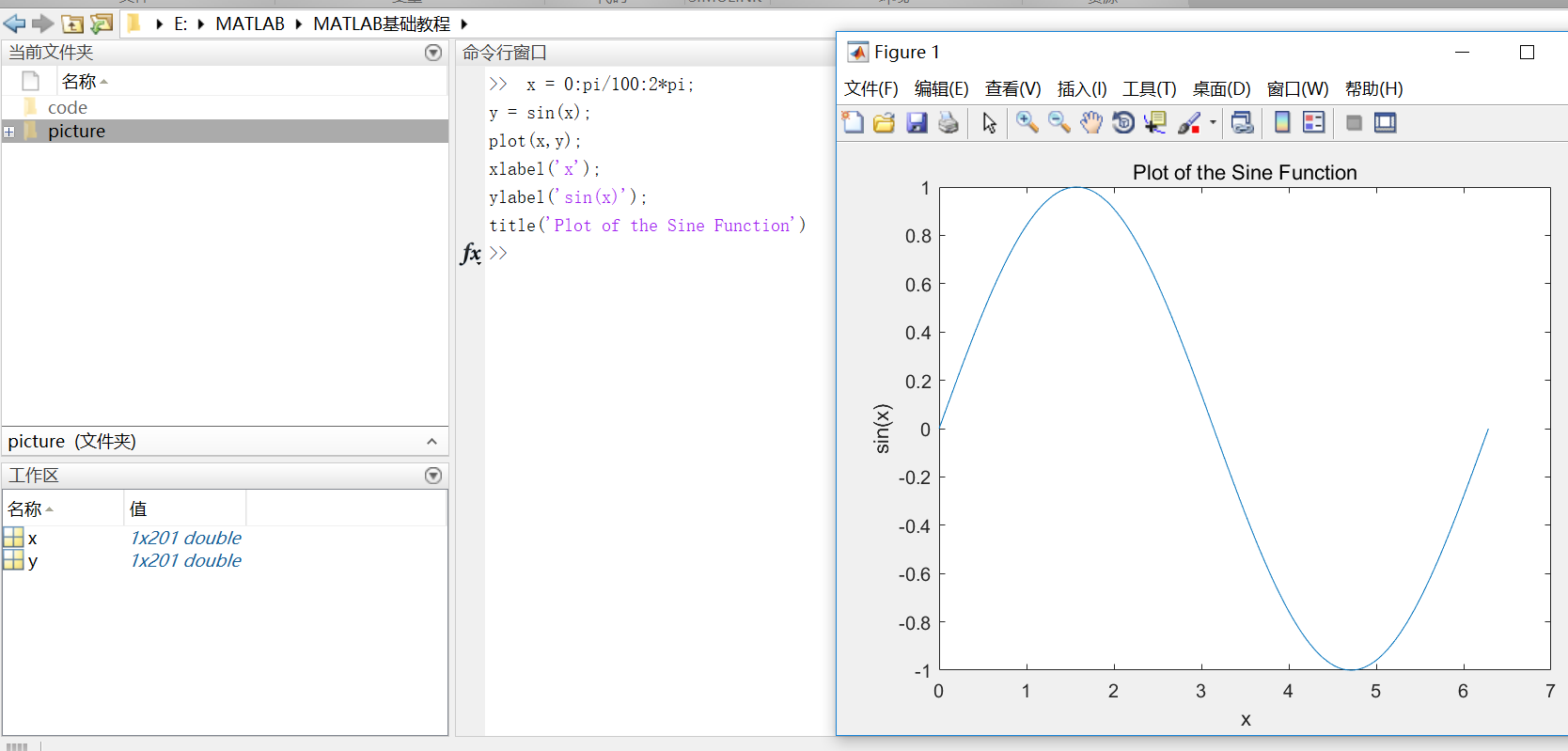（3）plot(x, y, ‘r–’)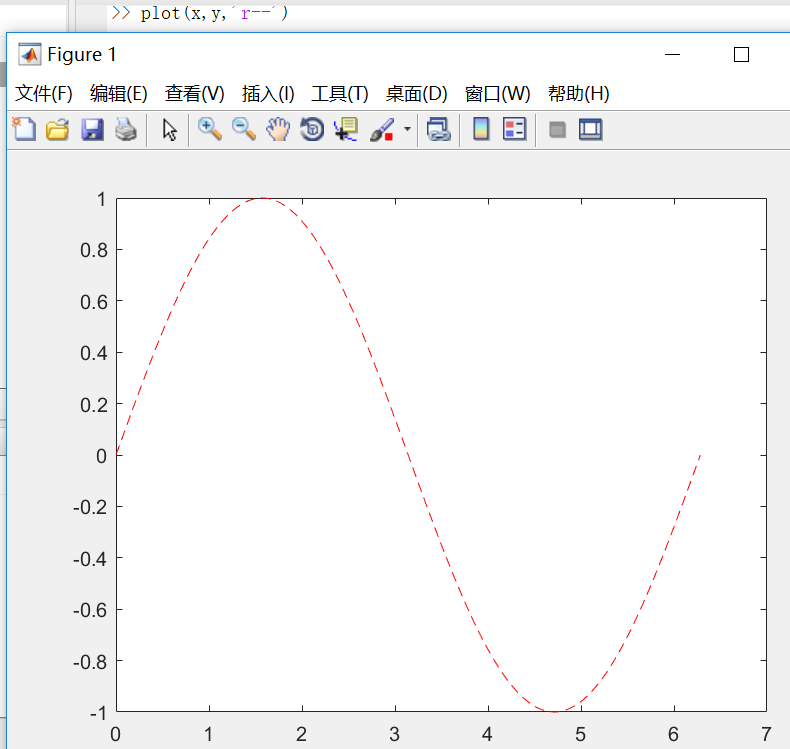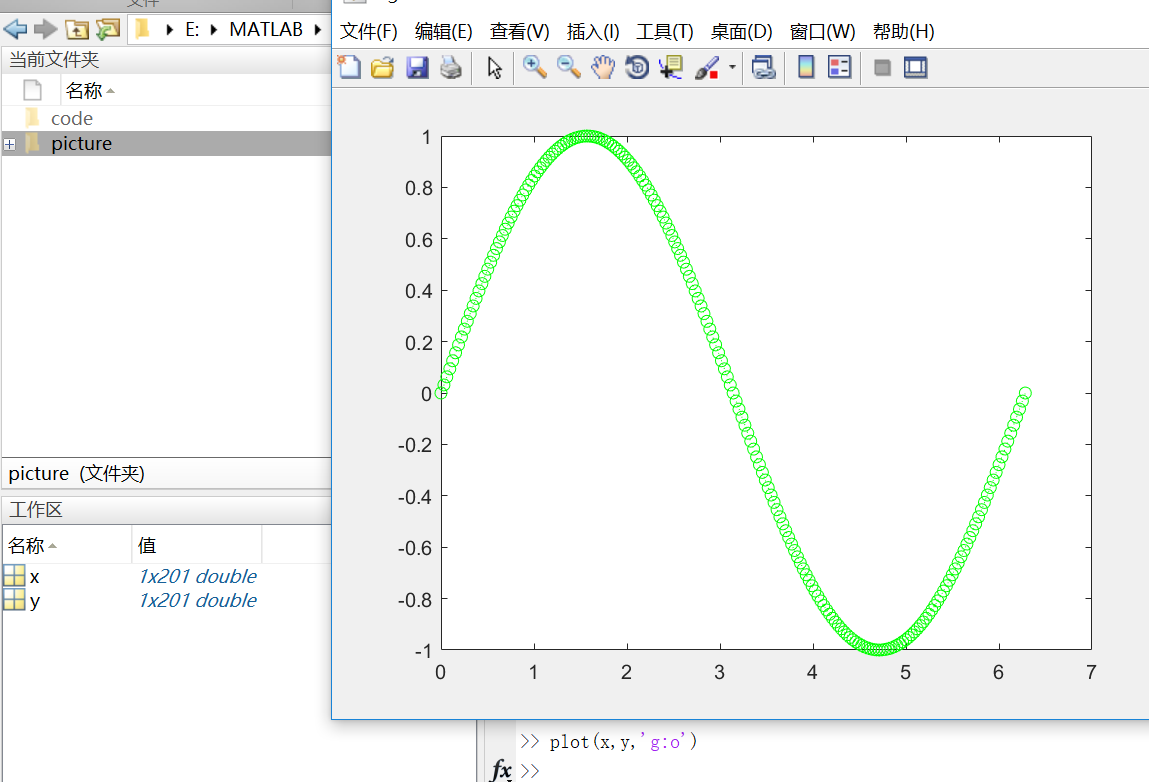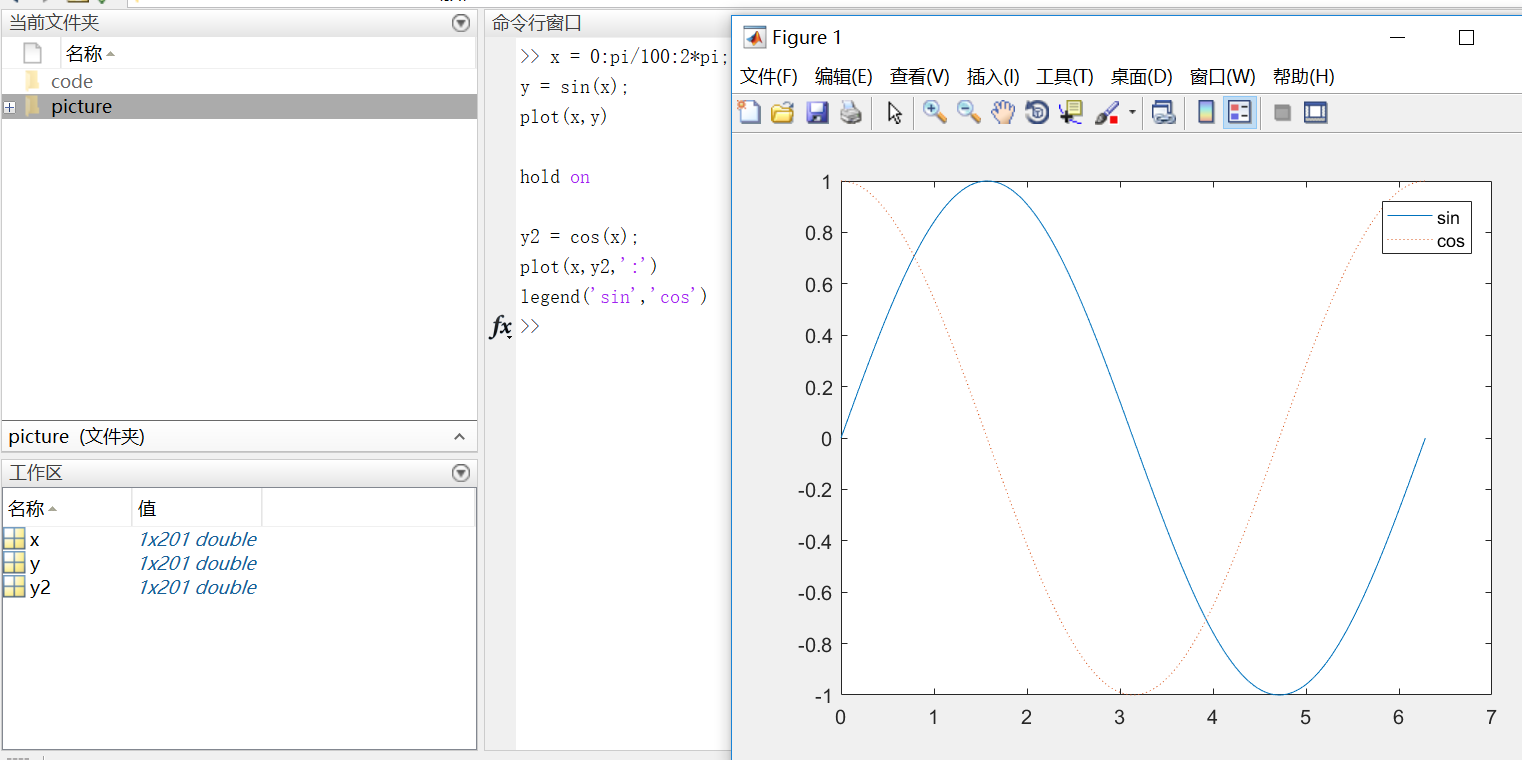• 3D图像

3D图像通常用有两个参数的函数来定义一个曲面，z = f(x,y) 。
对于z，首先使用meshgrid命令在函数定义域内创建若干(x,y)。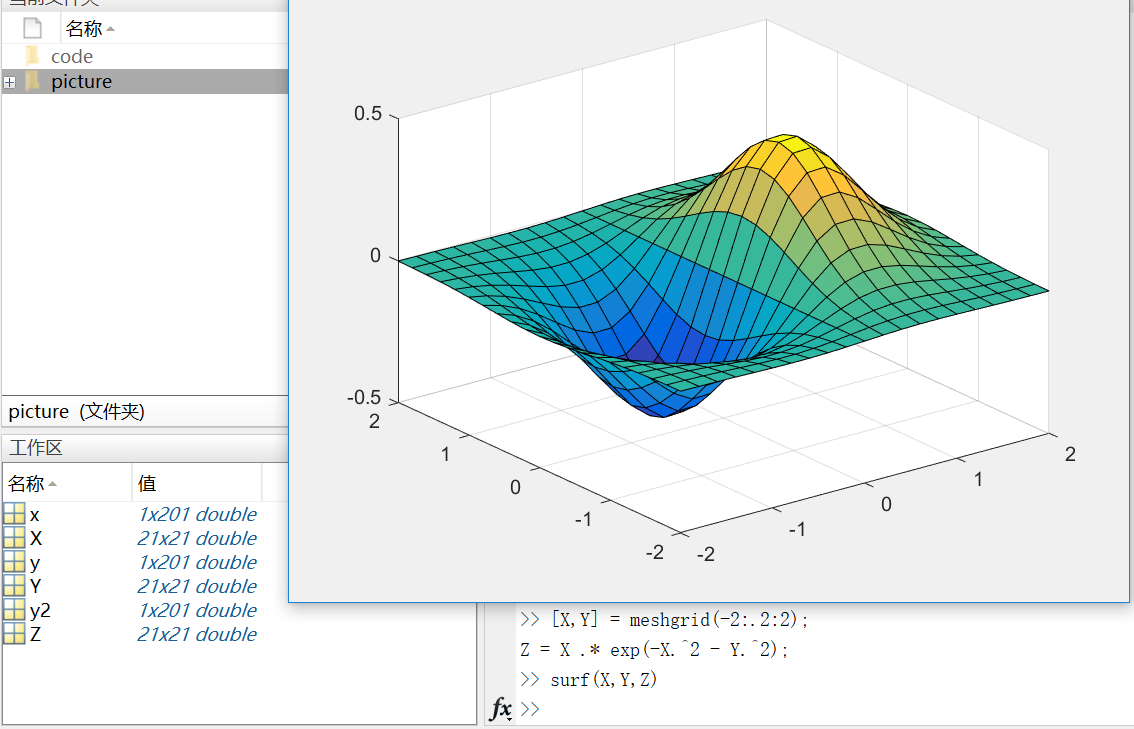(-2:.2:2)表示在-2到2的区间上以0.2的长度为间隔的向量；

• Subplots
你可以用subplot函数在同一窗口的不同区域显示多个图像。
subplots函数的前两个输入参数显示当前窗口每行每列的图像数量，第三个输入参数指定活动区域。例如，在图形窗口创建四个区域来分别输出图像。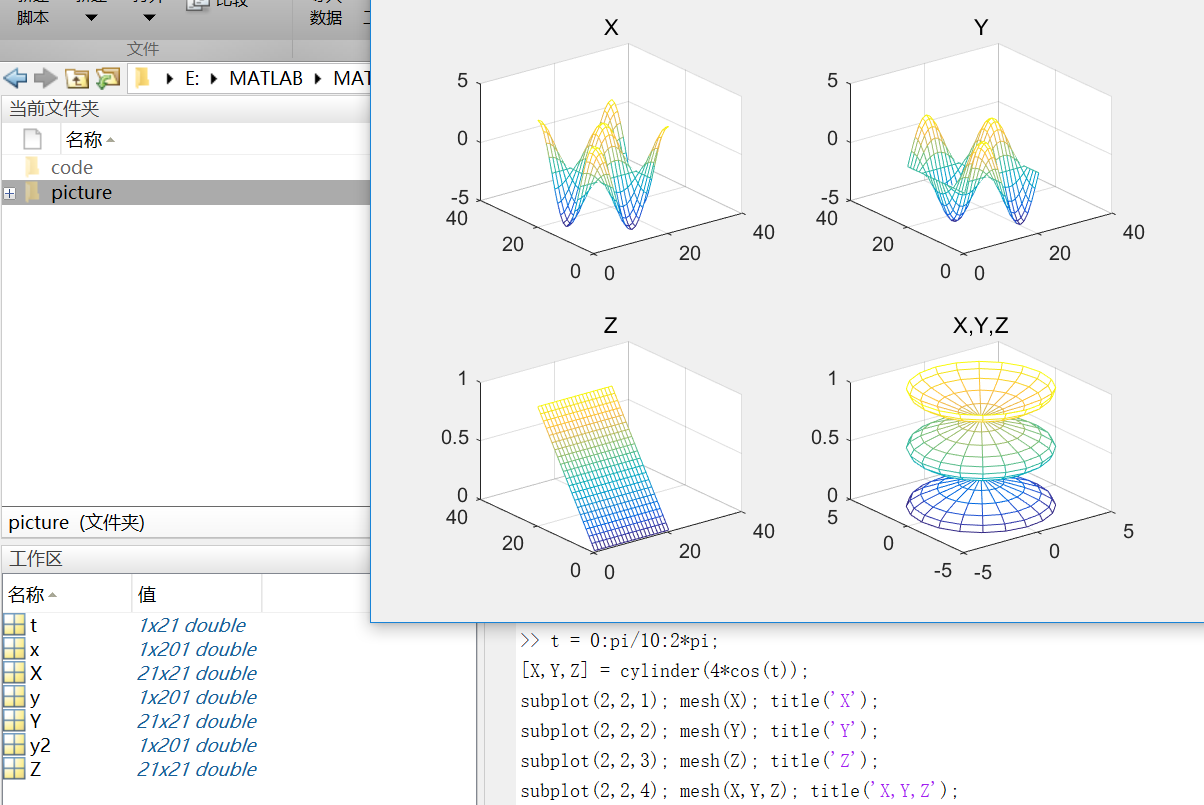mesh()生成由X，Y和Z指定的网线面，由C指定的颜色的三维网格图。

https://cn.mathworks.com/help/matlab/learn_matlab/scripts.html

• 示例脚本（从均匀分布中生成随机数据并计算平均值。绘制数据和平均值。）
用edit命令来创建脚本：
edit plotrand
这样就打开了一个叫plotrand.m的空文件，输入产生随机数向量的代码：
n = 50;
r = rand(n,1);
plot(r)

m = mean(r);
hold on
plot([0,n],[m,m])
hold off
title('Mean of Random Uniform Data')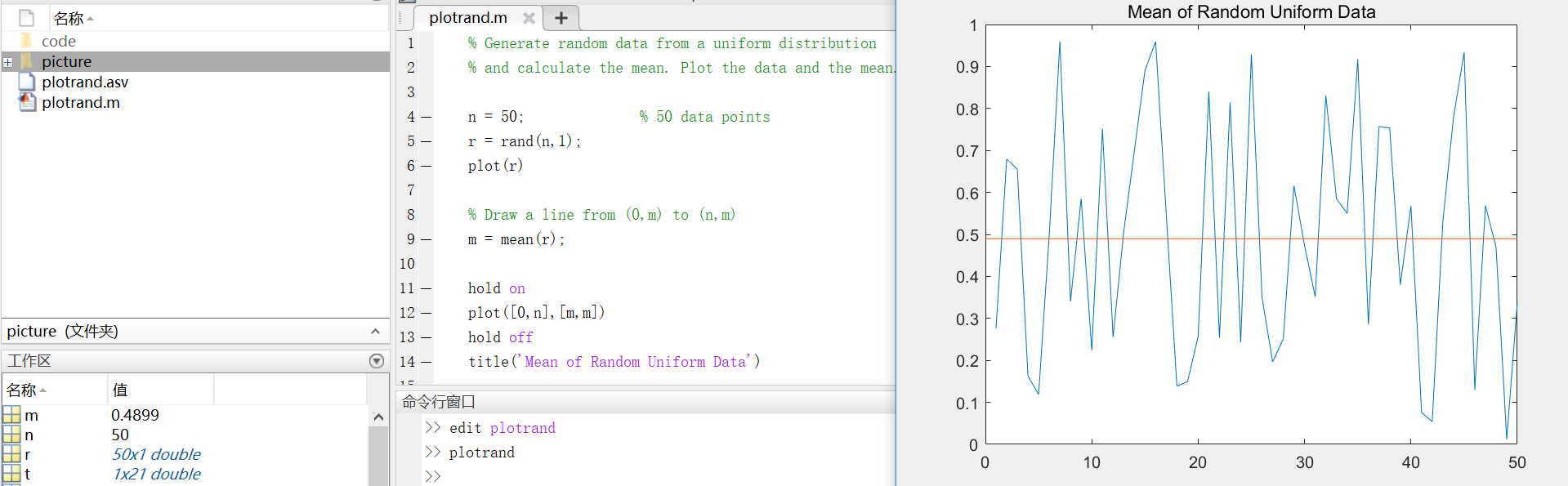• 循环和条件语句
在脚本中，可以循环使用部分代码，并且通过使用（for,while,if,switch等关键字）来执行这部分代码。
例如，创建一个叫calcmean.m的脚本，利用for循环计算五个随机样本的平均数和总平均数。
nsamples = 5;
npoints = 50;

for k = 1:nsamples
currentData = rand(npoints,1);
sampleMean(k) = mean(currentData);
end
overallMean = mean(sampleMean)

for k = 1:nsamples
iterationString = ['Iteration #',int2str(k)];
disp(iterationString)
currentData = rand(npoints,1);
sampleMean(k) = mean(currentData)
end
overallMean = mean(sampleMean)

calcmean

if overallMean < .49
disp('Mean is less than expected')
elseif overallMean > .51
disp('Mean is greater than expected')
else
disp('Mean is within the expected range')
end
• 脚本位置
MATLAB中，要运行脚本，文件必须位于当前文件夹或搜索路径中的文件夹中。
默认情况下，MATLAB安装程序创建的MATLAB文件夹位于搜索路径上。如果要在另一个文件夹中存储和运行程序，需要将其添加到搜索路径中：在当前文件夹浏览器中选择文件夹，右键单击，然后选择“添加到路径”。

https://cn.mathworks.com/help/matlab/learn_matlab/help.html

• 使用doc命令在单独的窗口中打开函数文档。

doc mean

• 键入左圆括号后暂停，MATLAB在命令窗口中就会显示函数提示（函数文档的语法部分）。

mean(

• 使用帮助命令后，在命令窗口中查看函数的简单用法概括。

help mean

04-10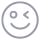插入表情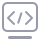代码片
06-2111万+
07-221万+
06-082万+
10-1119万+
07-081万+
07-2714万+
06-213万+
03-16226
09-133538
04-12
11-22
07-144万+
06-301466
01-061077
10-13729in_nocence

¥2 ¥4 ¥6 ¥10 ¥20余额支付 (余额：-- )扫码支付获取中扫码支付点击重新获取扫码支付1.余额是钱包充值的虚拟货币，按照1:1的比例进行支付金额的抵扣。
2.余额无法直接购买下载，可以购买VIP、C币套餐、付费专栏及课程。余额充值